Explore

Exercises

# Multiplication 2

This guided lesson builds upon third graders' understanding of multiplication in order to further develop fluency with this operation. The lesson first reinforces the various strategies for doing multiplication (for example, repeated addition), then teaches kids to apply those strategies within practical exercises. Designed by our team of teachers and curriculum experts, the goal is to provide numerous ways to conceptualize multiplication problems.
This lesson includes 5 printable learning activities.

## One Digit Multiplication with Missing Factors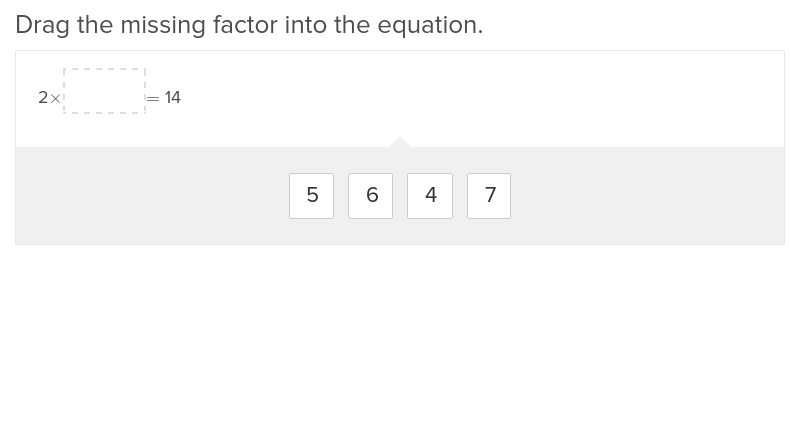If students are missing one digit in a multiplication problem, this exercise will help them walk through any challenges.

## One-Digit Multiplication Word Problems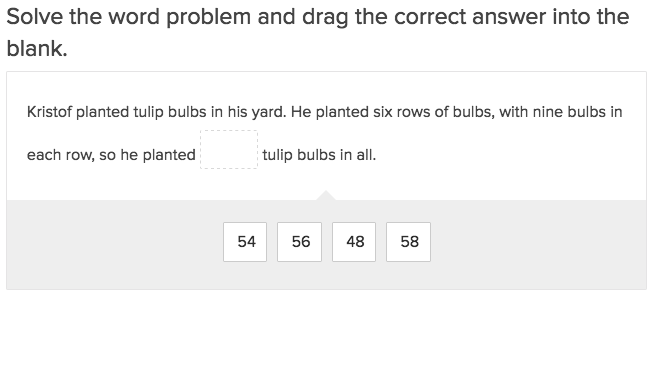If students are new to word problems, this exercise provides great one digit multiplication introductory tasks.

## Multiplication and the Distributive Property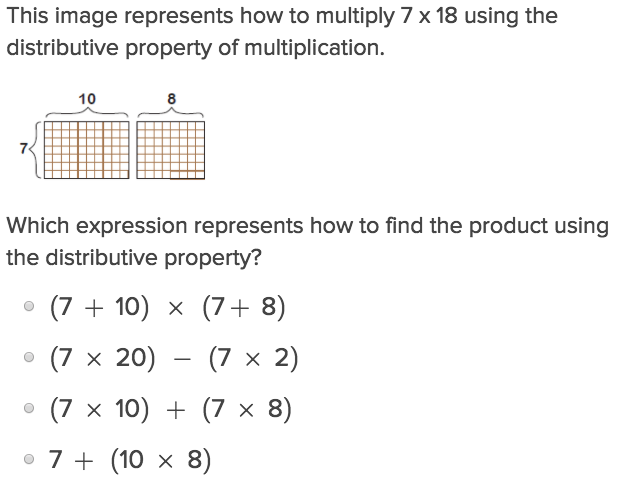As students grow more comfortable with the order of operations, they’ll need this exercise to introduce them to working with one digit distributive properties.

## Associative Property of Multiplication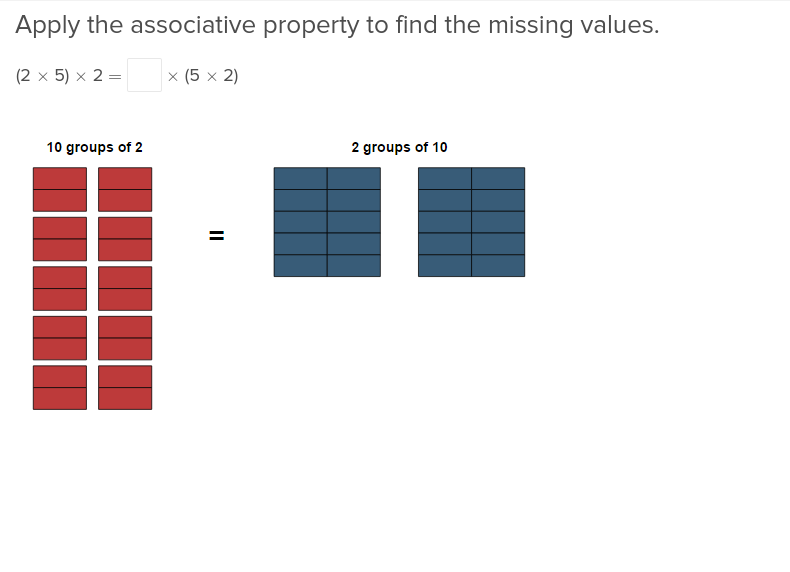Help your third grader master multiplication and the associative property with these exercises that walk through problems step by step.

## Multiplication and Two-Step Word Problems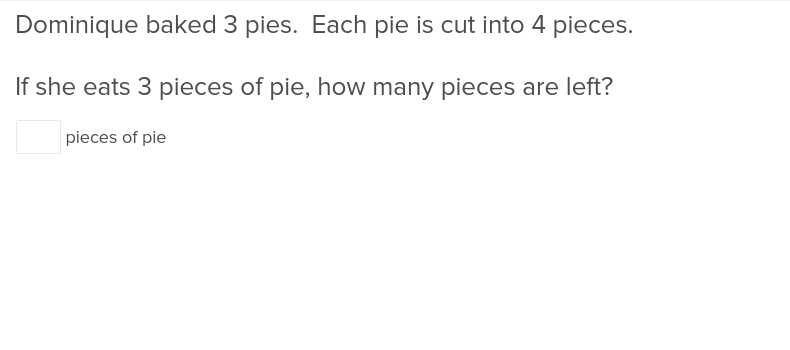Help your third grader apply their knowledge of math to real life situations with these exercises that include multi-step math problems.

## Multiply by 3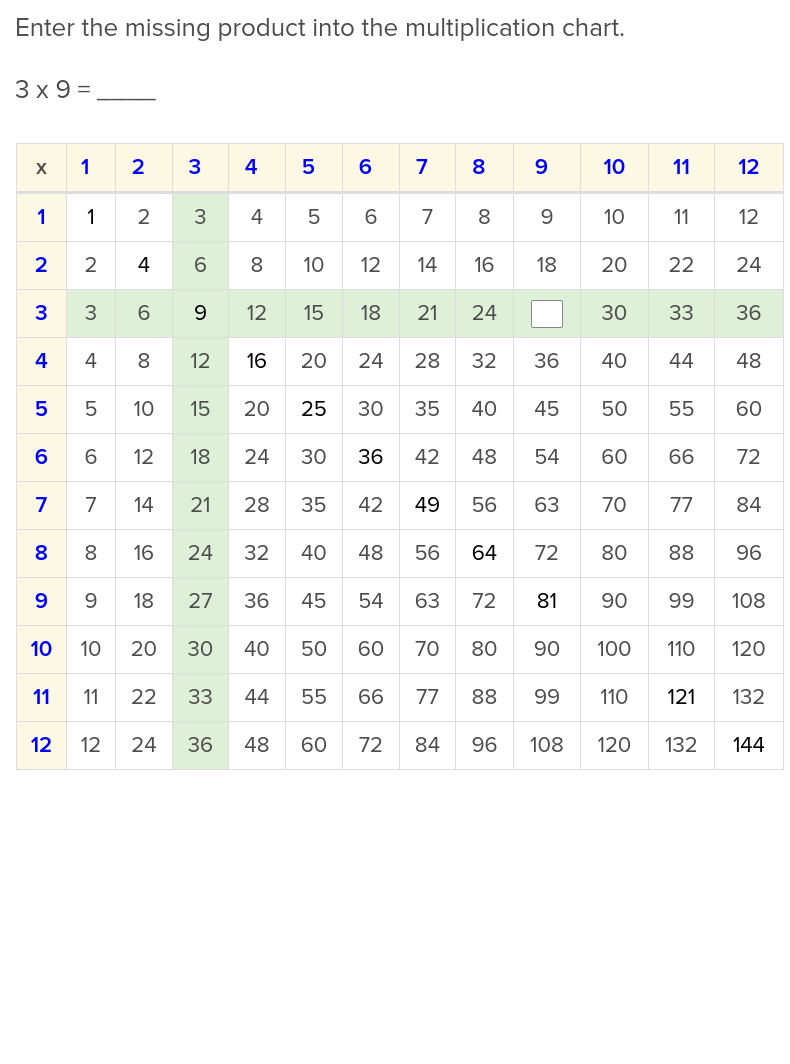Help students take their first steps into multiplication with this exercise on facts about multiplying 3s.

## Multiply by 4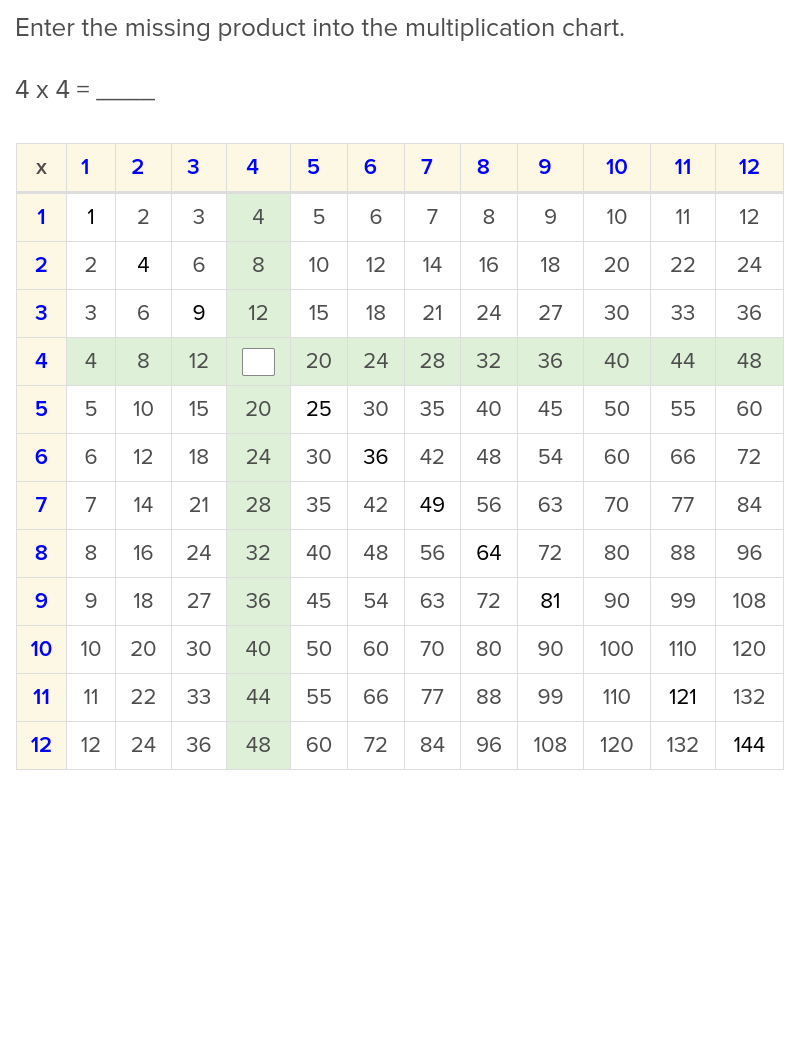When students are ready to take on a new challenge, this exercise on facts regarding multiplying 4s will stretch their skills.

## Multiply by 5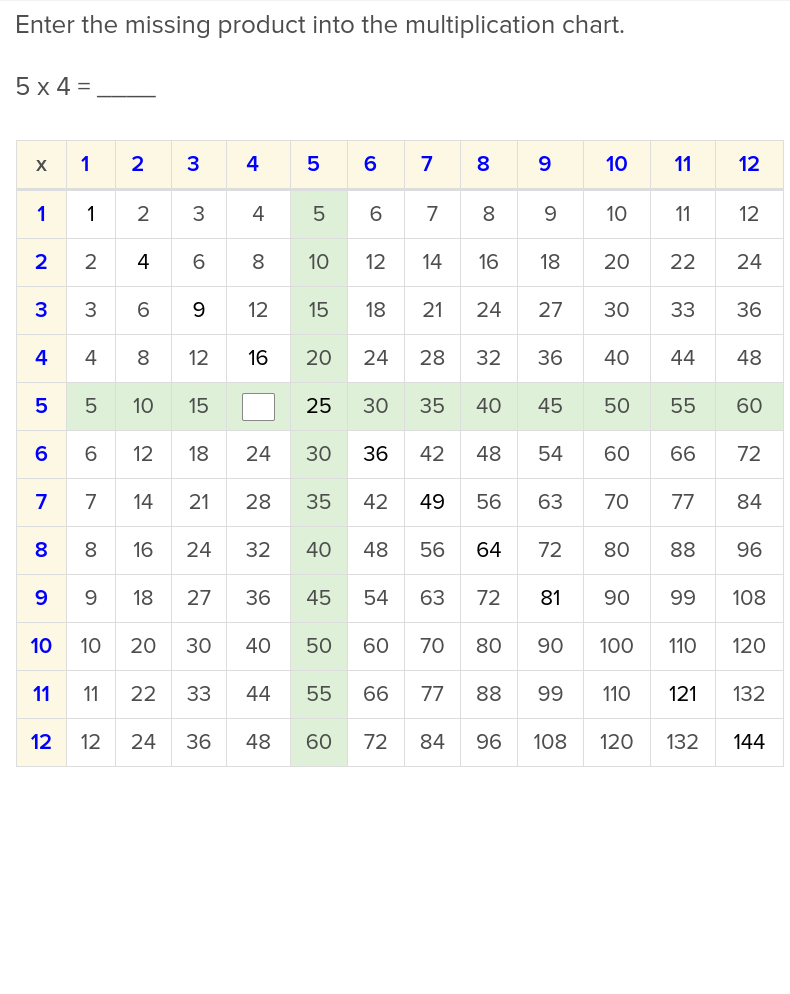Students will love studying their times tables once they have learned these facts about multiplying 5s.

## Multiply by 6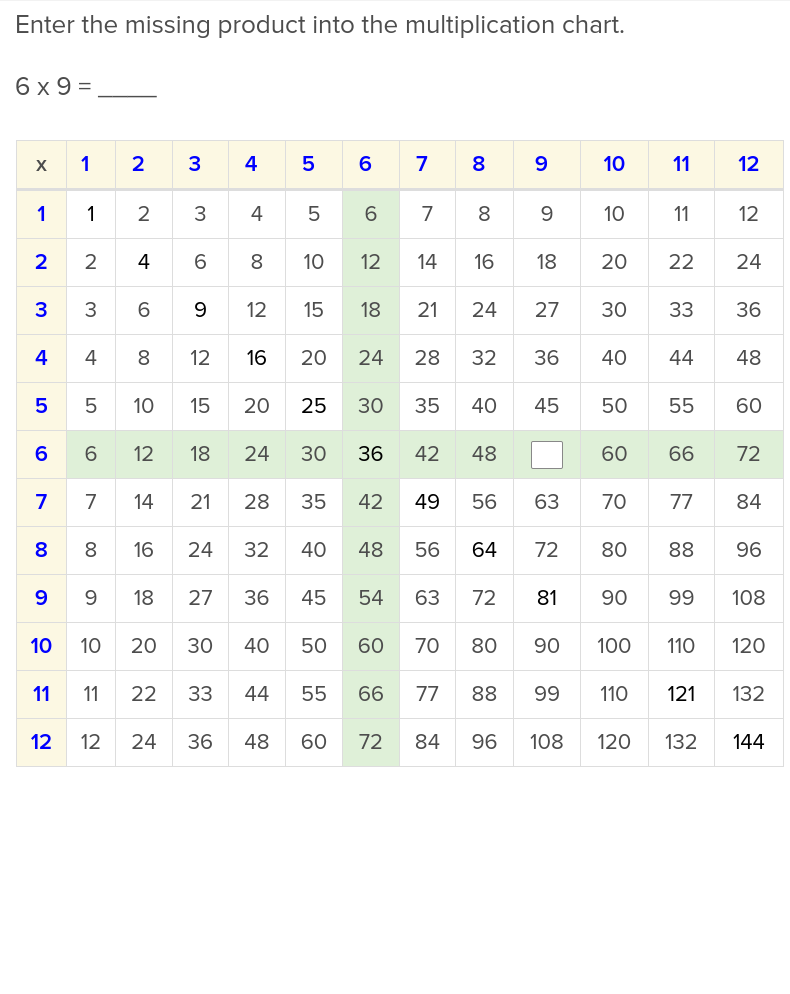Multiplying 6s will be much easier after students have learned some basic rules through this exercise.

## Multiply by 7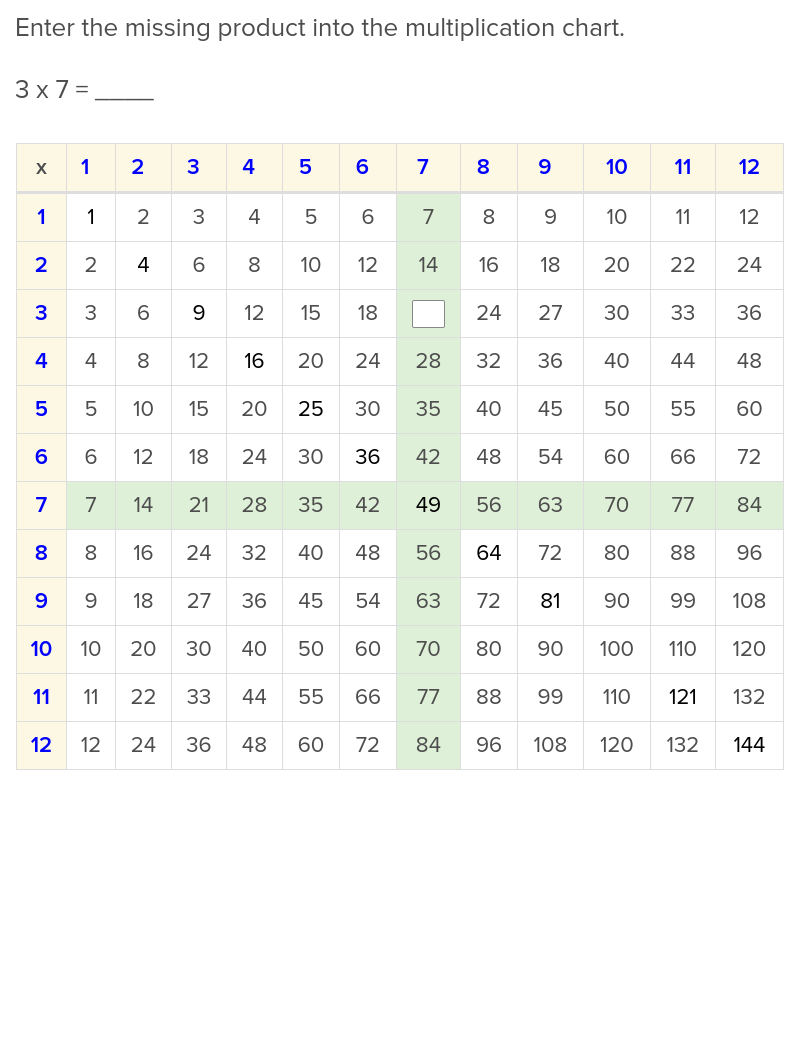Luckily for your students, they have this easy to use Education.com exercise on multiplying 7s.

## Multiply by 8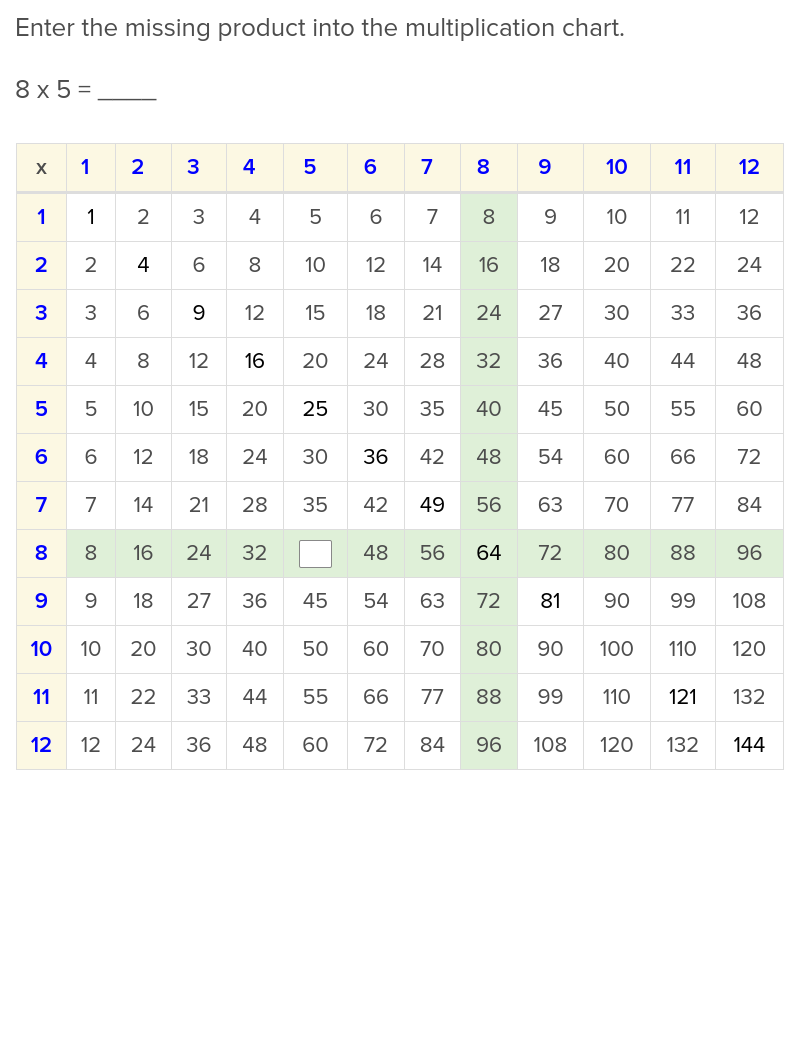Multiplying 8s is no longer a mystery to students who learn the facts taught in this exercise.

## Multiply by 9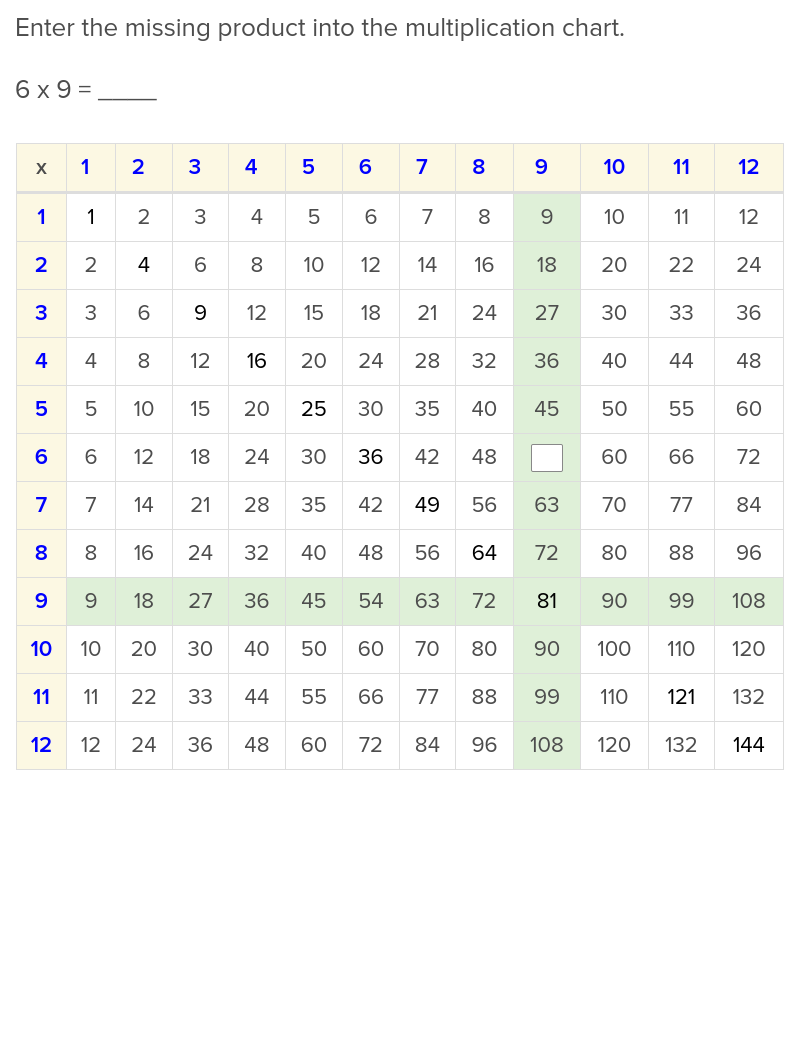You can teach your students the hand trick in conjunction with these facts about multiplying 9s.

Create new collection

0

### New Collection>

0 items

What could we do to improve Education.com?